Скачать презентацию Vertex Cover Dominating Set These slides are based

• Количество слайдов: 19Vertex Cover Dominating Set These slides are based on the Lecture Notes by David Mount for the course CMSC 451 at the University of Maryland. The Copyright notice is as follows: Copyright, David M. Mount, 2008 Dept. of Computer Science, University of Maryland, College Park, MD, 20742. These lecture notes were prepared by David Mount for the course CMSC 451, Design and Analysis of Computer Algorithms, at the University of Maryland. Permission to use, copy, modify, and distribute these notes for educational purposes and without fee is hereby granted, provided that this copyright notice appear in all copies.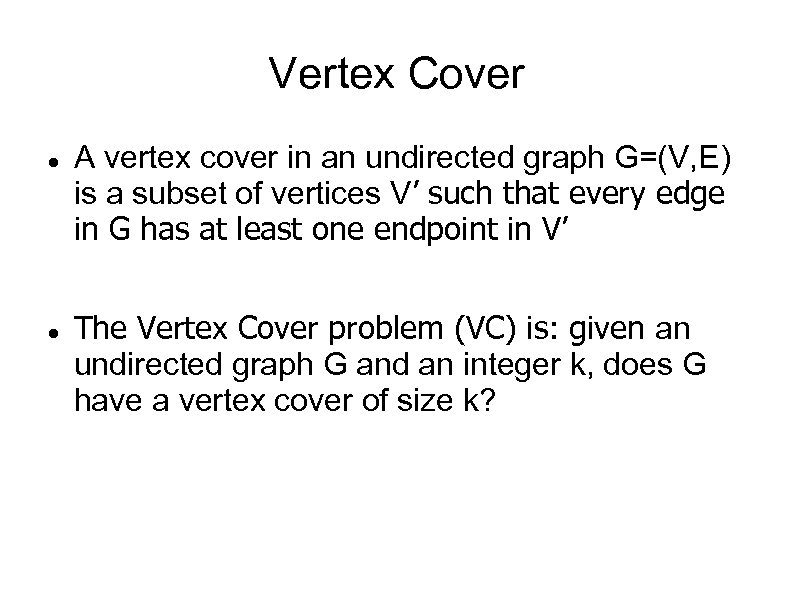Vertex Cover A vertex cover in an undirected graph G=(V, E) is a subset of vertices V’ such that every edge in G has at least one endpoint in V’ The Vertex Cover problem (VC) is: given an undirected graph G and an integer k, does G have a vertex cover of size k?Vertex cover E. g. useful in many servicing applications. For example, you have a compute network and a program that checks the integrity of the communication links. To save the space, instead of installing the program on every computer in the network, it suffices to install it on all the computers forming a vertex cover. From these nodes all the links can be tested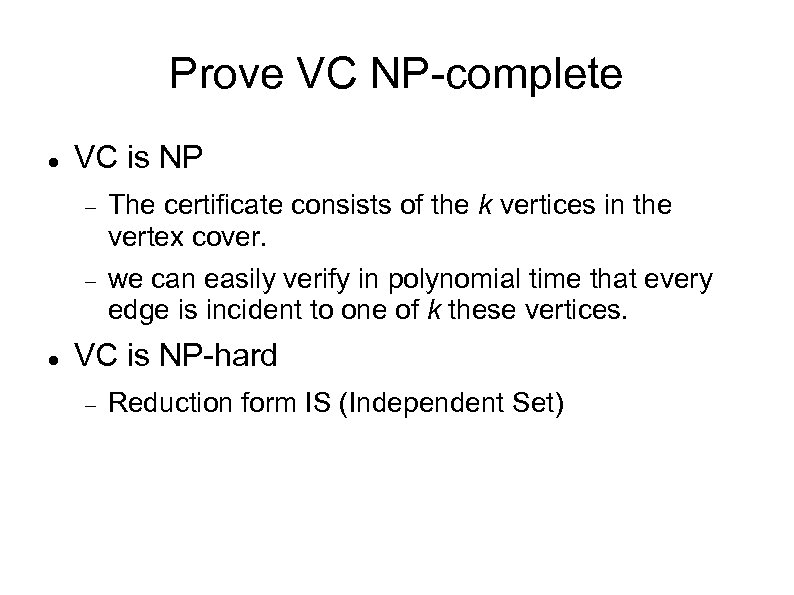Prove VC NP-complete VC is NP The certificate consists of the k vertices in the vertex cover. we can easily verify in polynomial time that every edge is incident to one of k these vertices. VC is NP-hard Reduction form IS (Independent Set)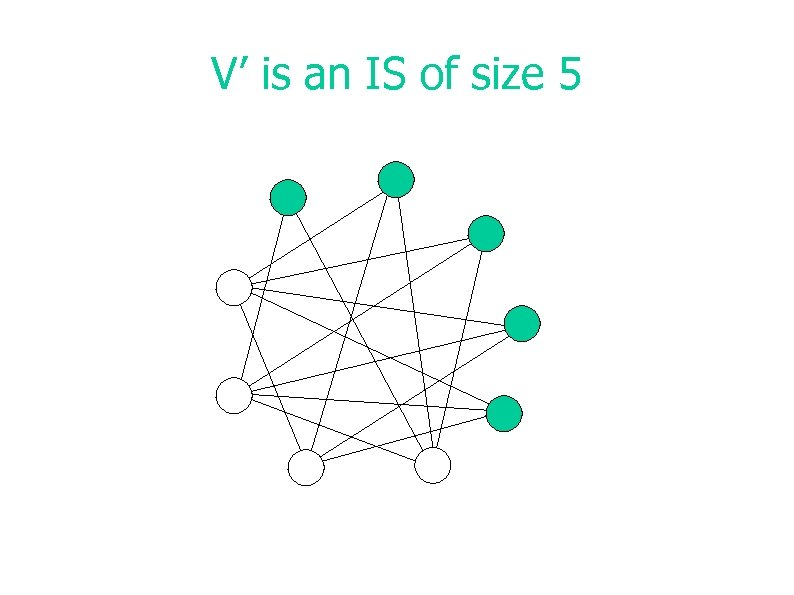V’ is an IS of size 5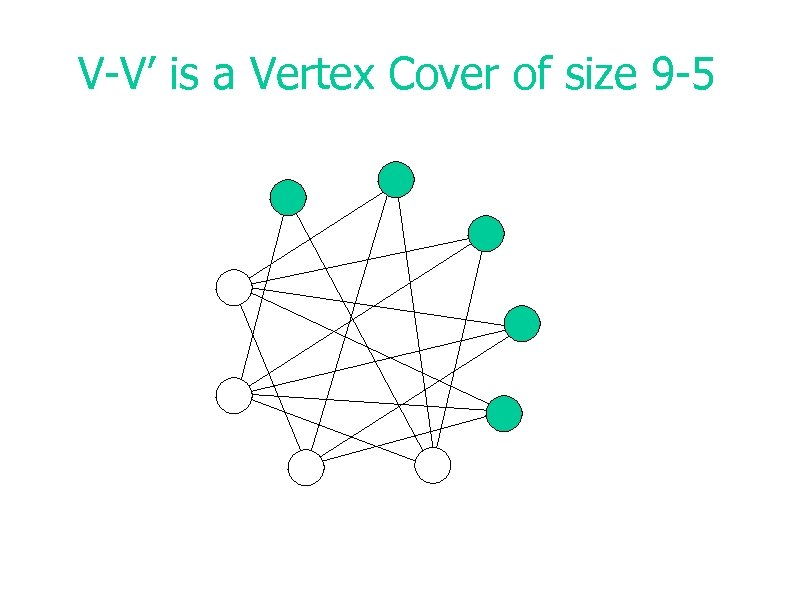V-V’ is a Vertex Cover of size 9 -5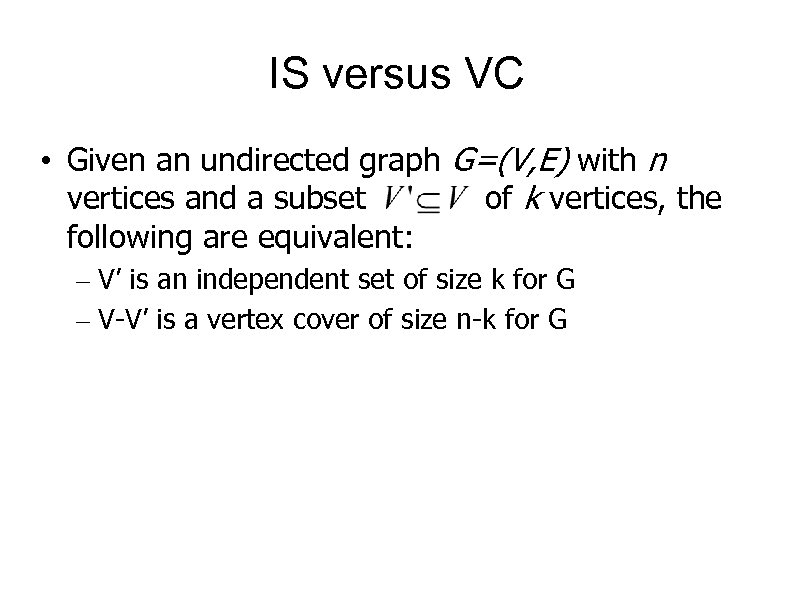IS versus VC • Given an undirected graph G=(V, E) with n vertices and a subset of k vertices, the following are equivalent: – V’ is an independent set of size k for G – V-V’ is a vertex cover of size n-k for G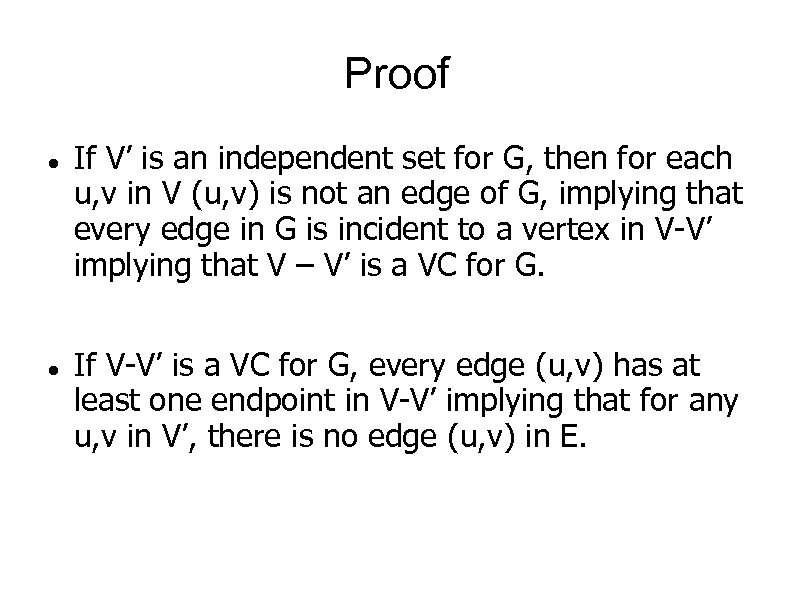Proof If V’ is an independent set for G, then for each u, v in V (u, v) is not an edge of G, implying that every edge in G is incident to a vertex in V-V’ implying that V – V’ is a VC for G. If V-V’ is a VC for G, every edge (u, v) has at least one endpoint in V-V’ implying that for any u, v in V’, there is no edge (u, v) in E.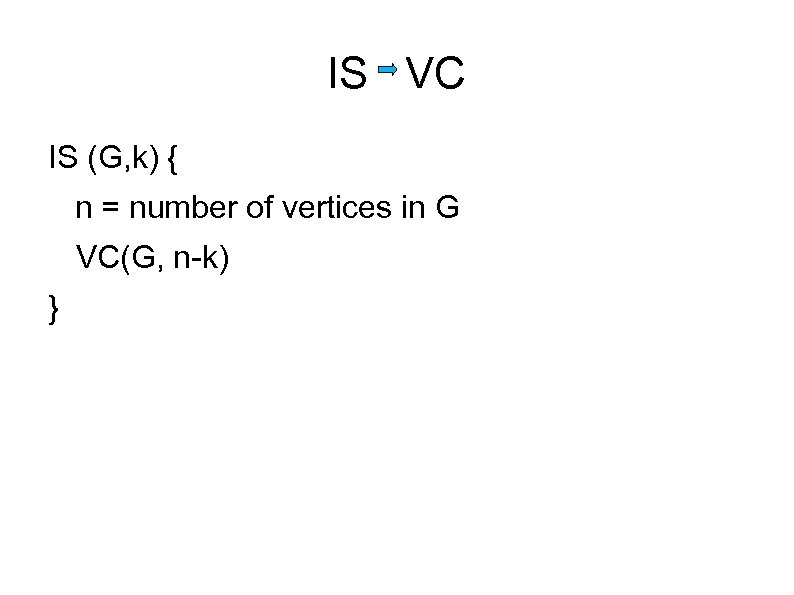IS VC IS (G, k) { n = number of vertices in G VC(G, n-k) }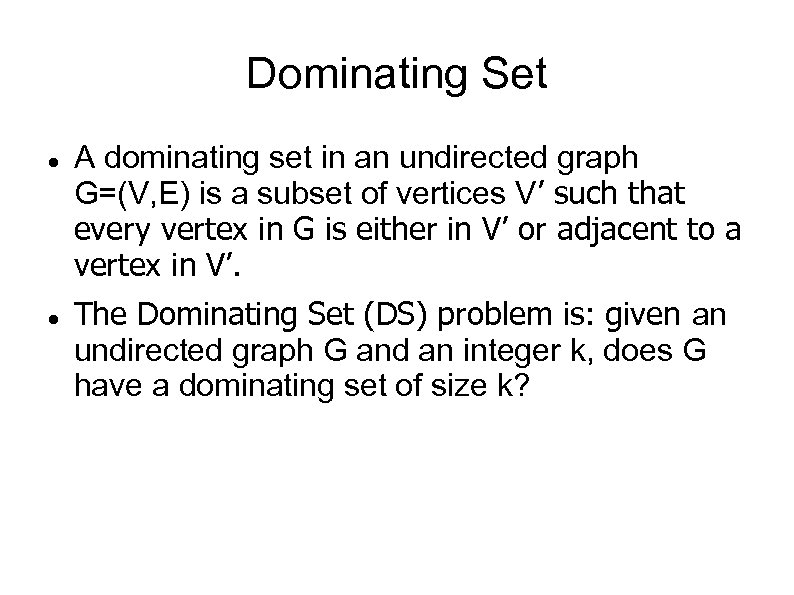Dominating Set A dominating set in an undirected graph G=(V, E) is a subset of vertices V’ such that every vertex in G is either in V’ or adjacent to a vertex in V’. The Dominating Set (DS) problem is: given an undirected graph G and an integer k, does G have a dominating set of size k?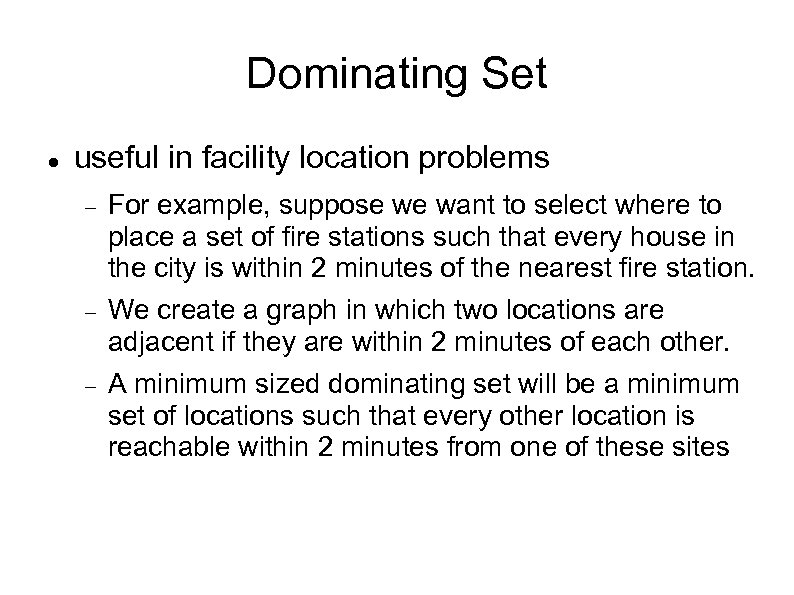Dominating Set useful in facility location problems For example, suppose we want to select where to place a set of fire stations such that every house in the city is within 2 minutes of the nearest fire station. We create a graph in which two locations are adjacent if they are within 2 minutes of each other. A minimum sized dominating set will be a minimum set of locations such that every other location is reachable within 2 minutes from one of these sites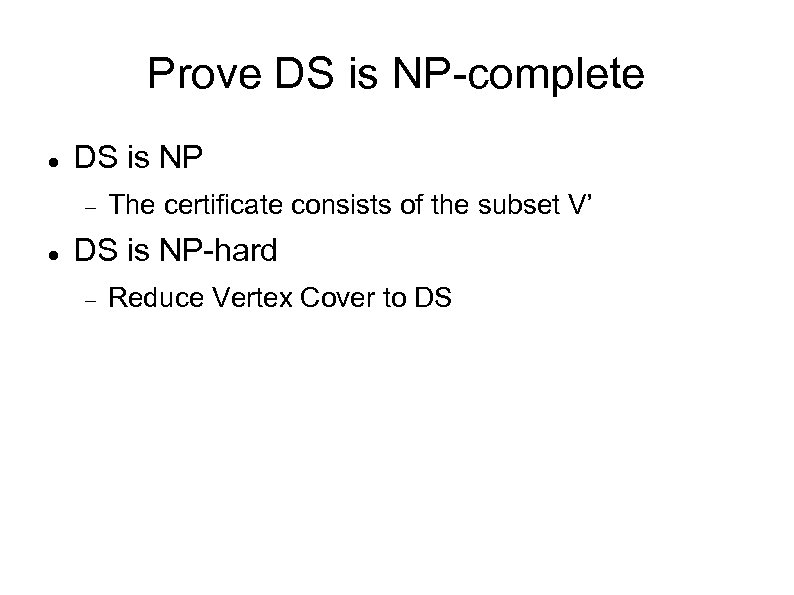Prove DS is NP-complete DS is NP The certificate consists of the subset V’ DS is NP-hard Reduce Vertex Cover to DS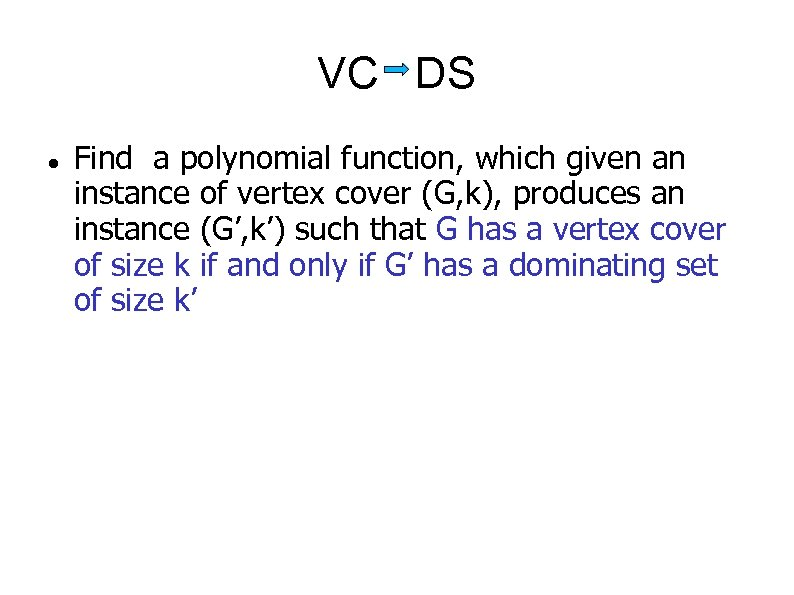VC DS Find a polynomial function, which given an instance of vertex cover (G, k), produces an instance (G’, k’) such that G has a vertex cover of size k if and only if G’ has a dominating set of size k’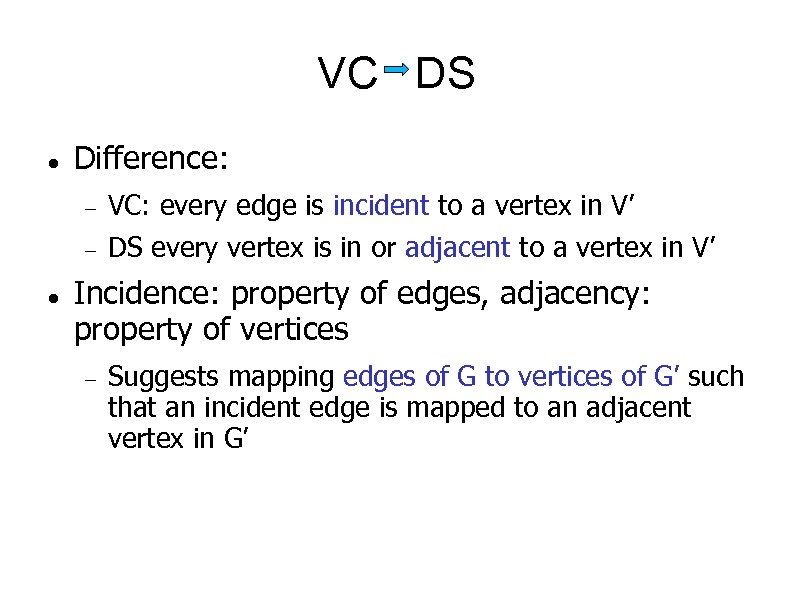VC DS Difference: VC: every edge is incident to a vertex in V’ DS every vertex is in or adjacent to a vertex in V’ Incidence: property of edges, adjacency: property of vertices Suggests mapping edges of G to vertices of G’ such that an incident edge is mapped to an adjacent vertex in G’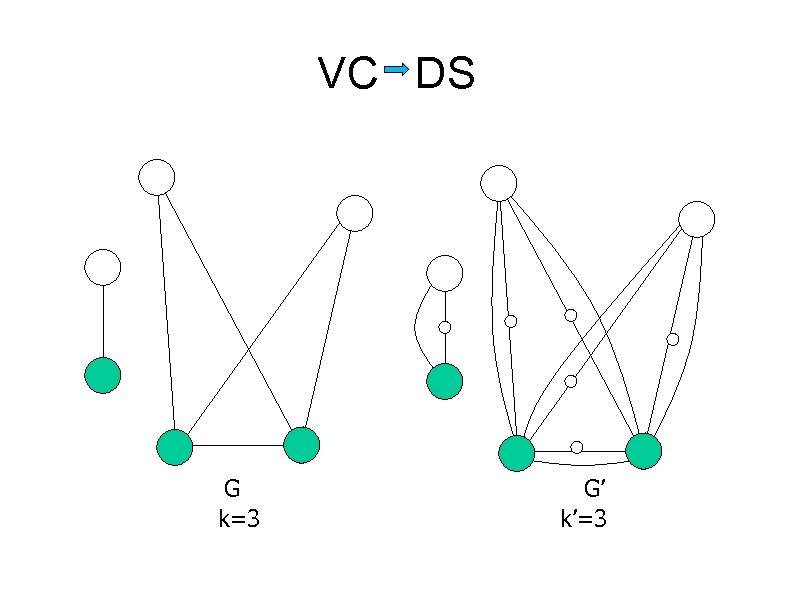VC DS G k=3 G’ k’=3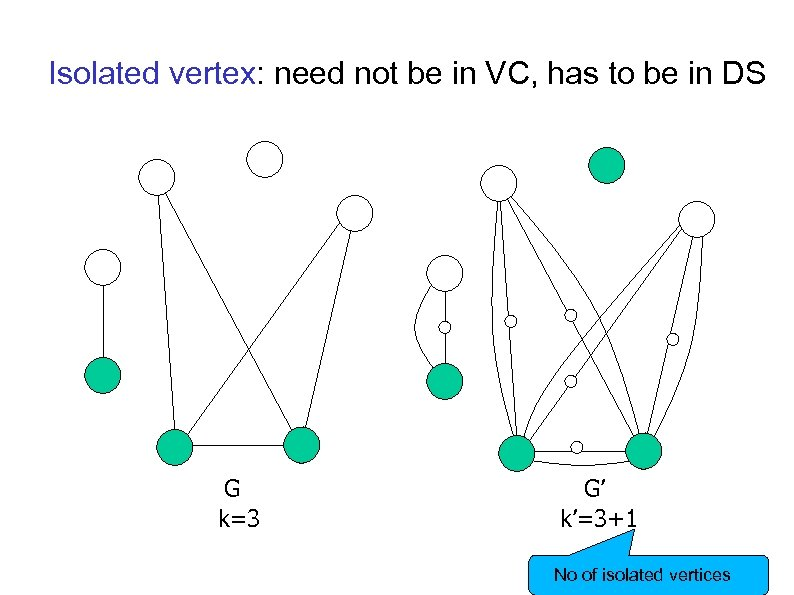Isolated vertex: need not be in VC, has to be in DS G k=3 G’ k’=3+1 No of isolated vertices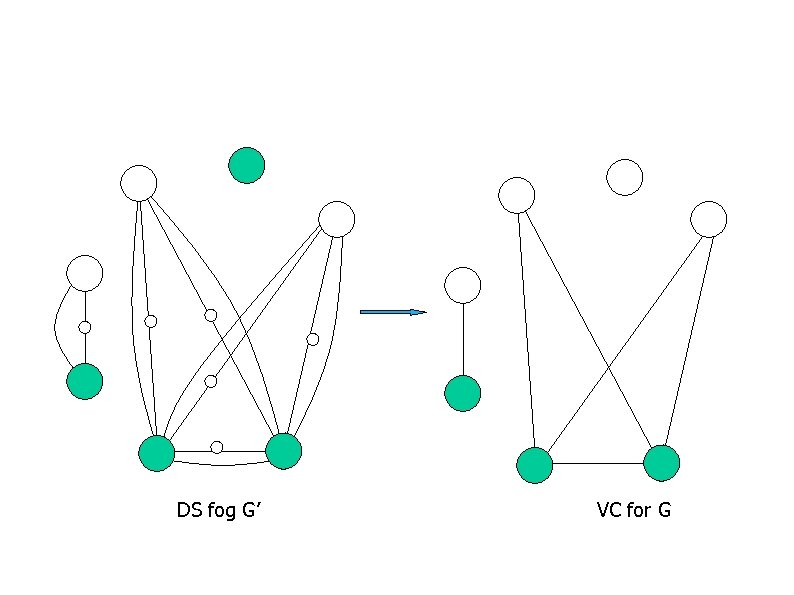DS fog G’ VC for G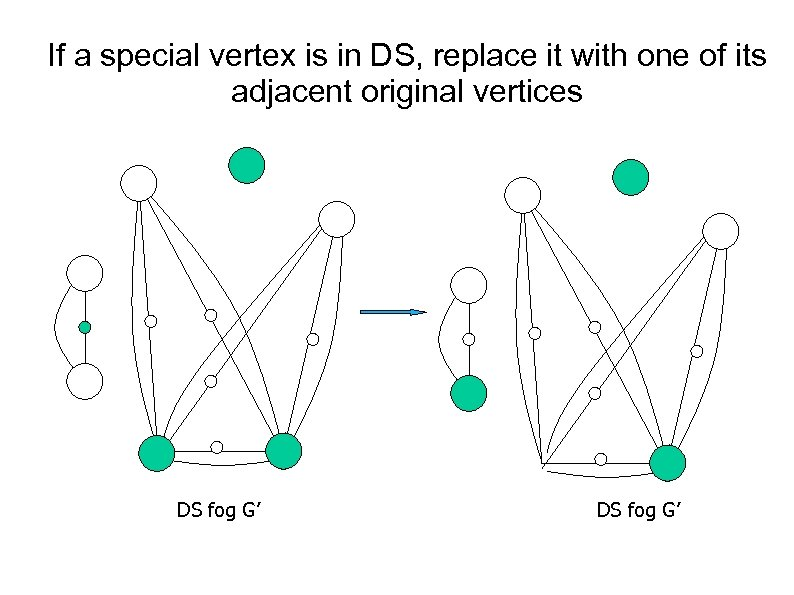If a special vertex is in DS, replace it with one of its adjacent original vertices DS fog G’DS fog G’ VC for G